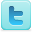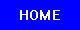﻿ Effects of Temperature on Surface Tension of Liquids
Effects of Temperature on Surface Tension of Liquids
K-12 Experiments & Background Information
For Science Labs, Lesson Plans, Class Activities & Science Fair Projects
For Elementary, Middle and High School Students & Teachers

Temperature and Surface Tension Experiments

• The Effect of Temperature on the Surface Tension of Water [View Experiment]
• Effect of Air at Various Temperatures on the Density and Surface Tension of Water and Alcohol [View Experiment]
• Variation of surface tension with temperature [View Experiment]
• The Effect of Temperature on the Surface Tension of Water [View Experiment]
• Density and Temperature Dependencies of Liquid Surface Tension [View Experiment]
• International Tables of the Surface Tension of Water [View Experiment]
• The surface tension of liquids measured with the stalagmometer [View Experiment]
• Surface tension by the ring method (Du Nouy method) [View Experiment]
• Investigate the effects of types of liquid and temperature on surface tension of water. [View Experiment]
• Temperature Effects on Surface Tension [View Experiment]
• Determine the surface tension of liquids against air by the method of capillary rise. [View Experiment]
• Measuring surface tension of binary mixtures of water + alcohols from the diffraction pattern of surface ripples [View Experiment]
• Surface Tension Lab: Investigate the property of the surface tension of water and compare water’s surface tension with two other liquids, oil and soapy water.[View Experiment]
Effects of Temperature on Surface Tension of Liquids

## Definition

Surface tension is a property of the surface of a liquid that allows it to resist an external force.

## Effects of Temperature on Surface Tension

Surface tension is dependent on temperature. For that reason, when a value is given for the surface tension of an interface, temperature must be explicitly stated. The general trend is that surface tension decreases with the increase of temperature, reaching a value of 0 at the critical temperature. For further details see the Eötvös rule below. There are only empirical equations to relate surface tension and temperature:

• Eötvös:
•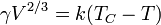Here V is the molar volume of that substance, TC is the critical temperature and k is a constant valid for almost all substances. A typical value is k = 2.1 x 10−7 [J K−1 mol-2/3]. For water one can further use V = 18 ml/mol and TC = 374°C.

A variant on Eötvös is described by Ramay and Shields: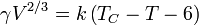where the temperature offset of 6 kelvins provides the formula with a better fit to reality at lower temperatures.

• Guggenheim-Katayama: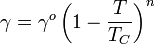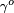is a constant for each liquid and n is an empirical factor, whose value is 11/9 for organic liquids. This equation was also proposed by van der Waals, who further proposed that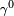could be given by the expression,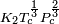, where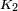is a universal constant for all liquids, andis the critical pressure of the liquid (although later experiments foundto vary to some degree from one liquid to another).

Both Guggenheim-Katayama and Eötvös take into account the fact that surface tension reaches 0 at the critical temperature, whereas Ramay and Shields fails to match reality at this endpoint.

## The Eötvös Rule

The Eötvös rule, named after the Hungarian physicist Loránd (Roland) Eötvös (1848–1919) enables the prediction of the surface tension of an arbitrary liquid pure substance at all temperatures. The density, molar mass and the critical temperature of the liquid have to be known. At the critical point the surface tension is zero.

The first assumption of the Eötvös rule is:

1. The surface tension is a linear function of the temperature.

This assumption is approximately fulfilled for most known liquids. When plotting the surface tension versus the temperature a fairly straight line can be seen which has a surface tension of zero at the critical temperature.

The Eötvös rule also gives a relation of the surface tension behaviour of different liquids in respect to each other:

2. The temperature dependence of the surface tension can be plotted for all liquids in a way that the data collapses to a single master curve. To do so either the molar mass, the density, or the molar volume of the corresponding liquid has to be known.

Source: Wikipedia (All text is available under the terms of the GNU Free Documentation License and Creative Commons Attribution-ShareAlike License.)

Science Fair Projects Resources
Physics Science Fair Books